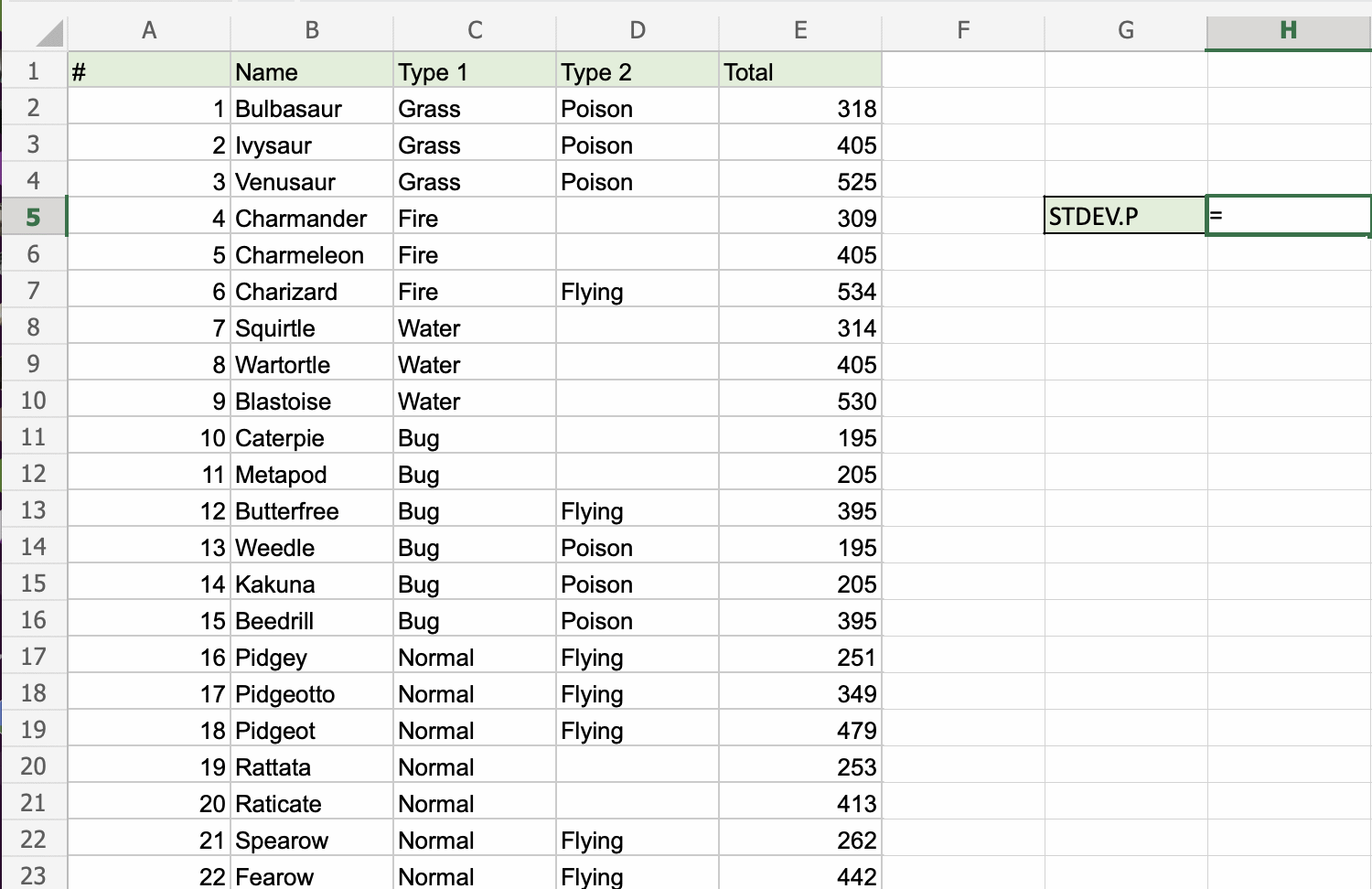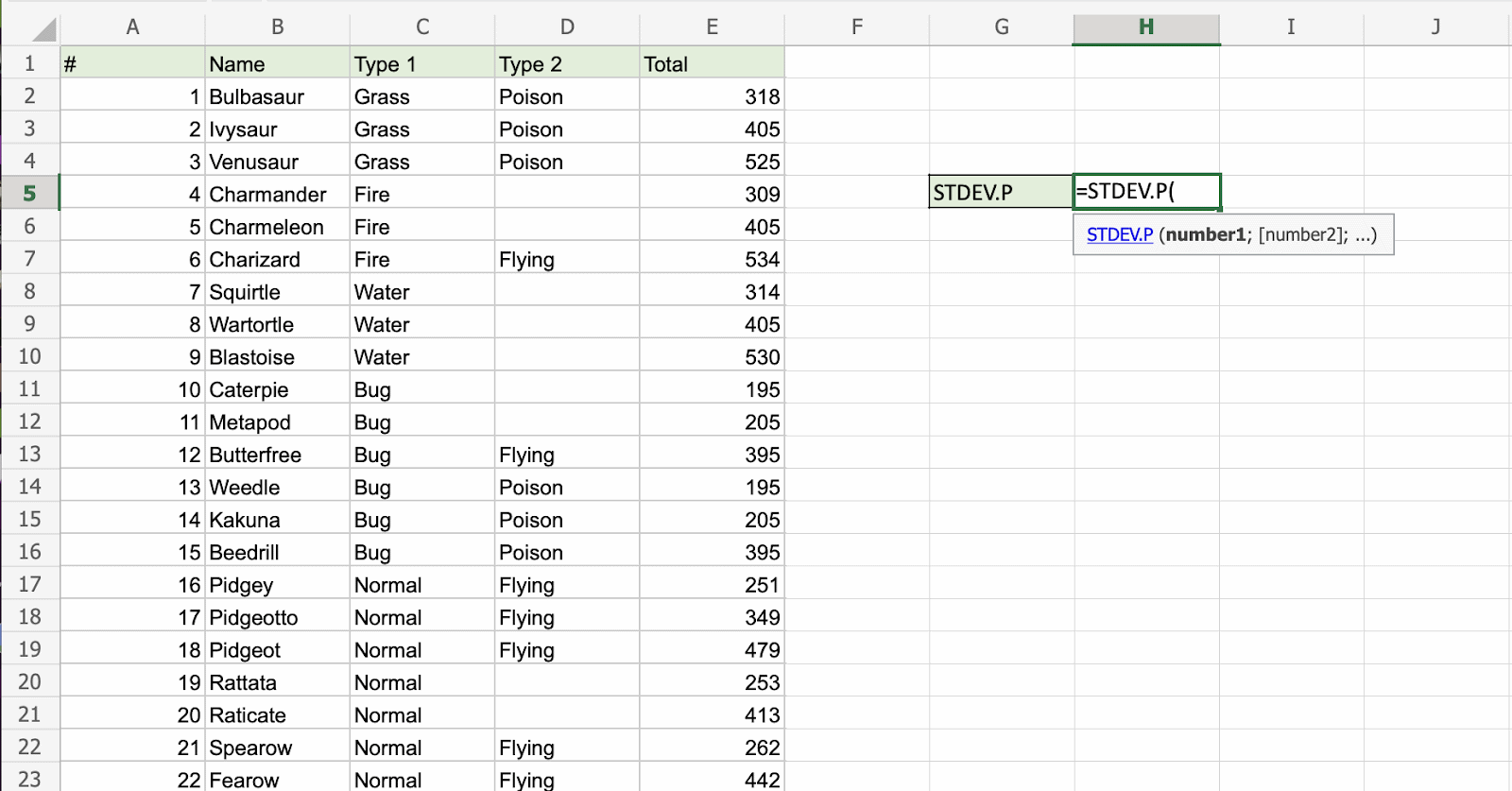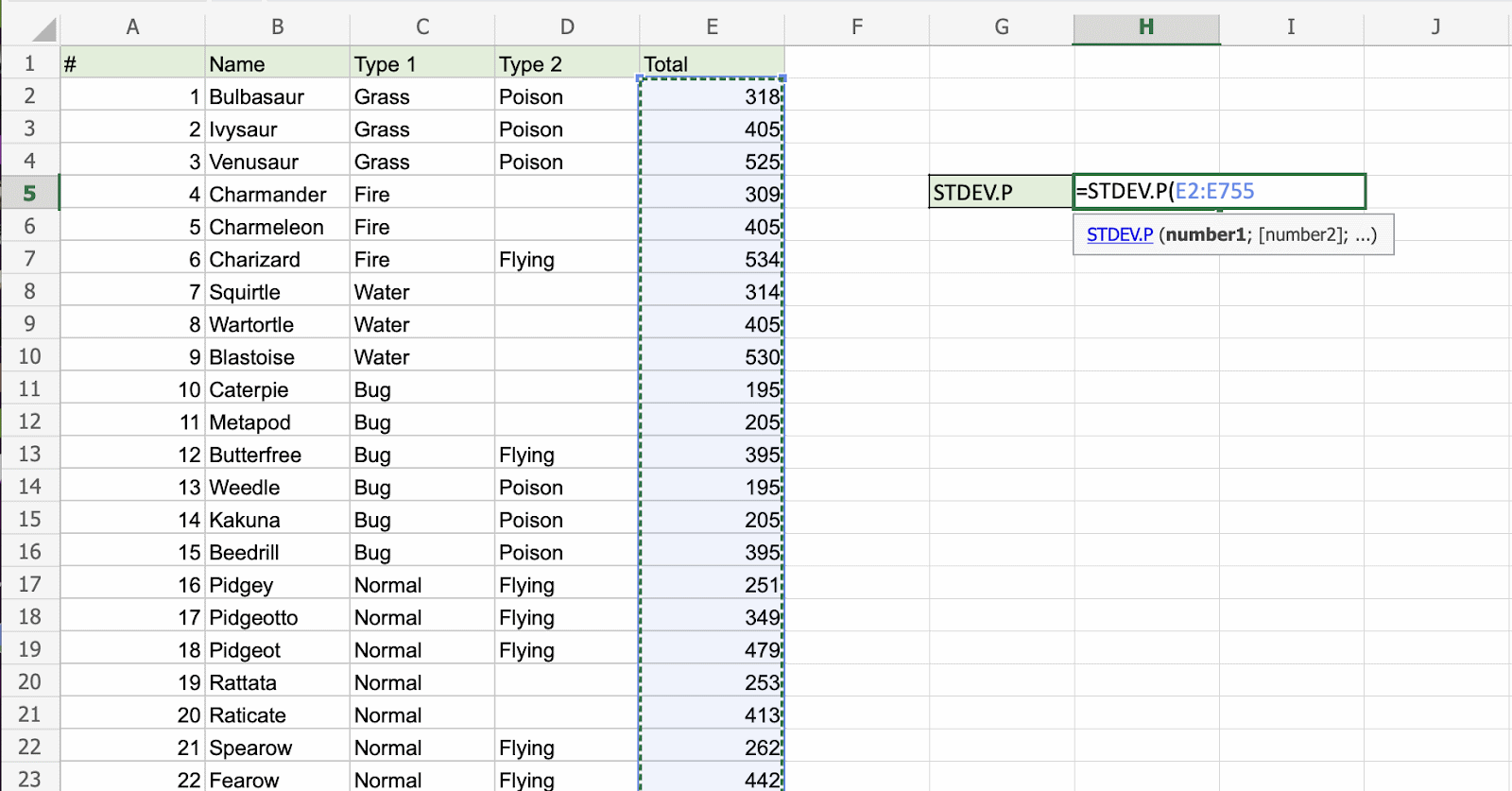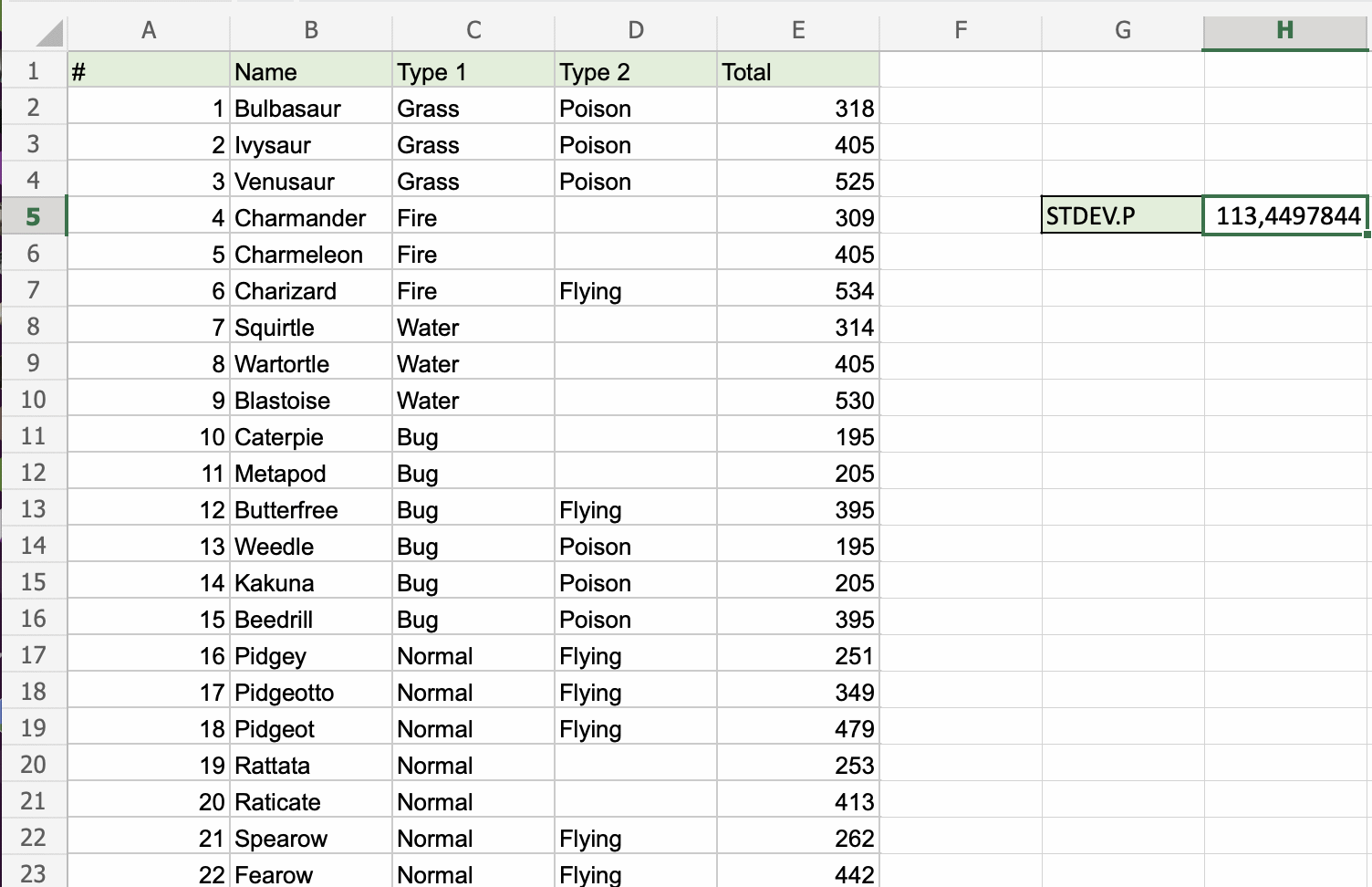# Excel STDEV.P Function

## STDEV.P Function

The STDEV.P function is a premade function in Excel, which calculates the Standard Deviation (Std) for the entire population.

It is typed `=STDEV.P`

Note: This function ignores cells with text and logic.

Note: Standard deviation (σ) measures how far a 'typical' observation is from the average of the data (μ). You can read more about standard deviation in our Statistics - Standard Deviation Tutorial.

Tip: There is another function called STDEV.S that can be used if you have the data for a sample.

How to use the `=STDEV.P` function:

1. Select a cell (`H5`)
2. Type `=STDEV.P`
3. Double click the STDEV.P command
4. Select a range (`E2:E755`), including all Pokemons
5. Hit enter

Let's have a look at an example!

Find the Standard Deviation of Pokemon total stats in the range `E2:E755`:The picture is not showing all rows. The range is `E2:E755` (753 rows).The STDEV.P function has successfully returned the Standard Deviation for the whole population as `113,4497844`.